## Converting Dates to Fiscal Quarters and Years

This post will guide you how to convert dates to fiscal quarters or years in Excel. How do I get fiscal quarter from a given date in Excel. How to calculate the fiscal year from a date in Excel. How to convert date to fiscal year or month with a formula in Excel.

If  you have a list of data in range B1:B5 which contain dates, and you want to calculate the fiscal quarters and years in your worksheet, how to achieve it. You can refer to the following introduction to achieve the result.

Assuming that the start month of the fiscal year in your company is April.

## Converting Dates to Fiscal Quarters

To calculate one date to a Fiscal quarters in your worksheet, you can use a formula based on the CHOOSE function and the MONTH function. Just like this:

`=CHOOSE(MONTH(B1),4,4,4,1,1,1,2,2,2,3,3,3)`

Select Cell C1, and type this formula into it, and press Enter key in your keyboard, and then drag the AutoFill Handle over other cells to apply this formula.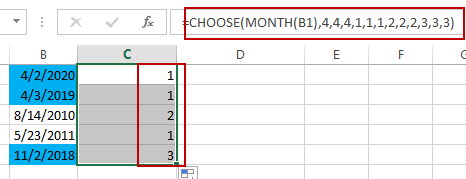The Fiscal quarters have been calculated.

## Converting Dates to Fiscal Years

To convert dates in cells to Fiscal years, you can create a formula based on the YEAR function and the MONTH function. Just like the following formula:

`=YEAR(B1)+(MONTH(B1)>=4)`

Type this formula in Cell C1, and press Enter key, and then drag the AutoFill Handle over other cells to apply this formula.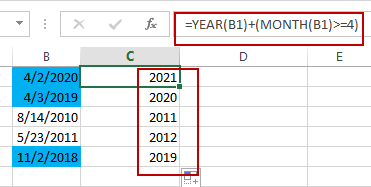The Fiscal Years have been converted successfully in your worksheet.

### Related Functions

• Excel Choose Function
The Excel CHOOSE function returns a value from a list of values. The CHOOSE function is a build-in function in Microsoft Excel and it is categorized as a Lookup and Reference Function.The syntax of the CHOOSE function is as below:=CHOOSE (index_num, value1,[value2],…)…
• Excel YEAR function
The Excel YEAR function returns a four-digit year from a given date value, the year is returned as an integer ranging from 1900 to 9999. The syntax of the YEAR function is as below:=YEAR (serial_number)…
• Excel MONTH function
The Excel MONTH function returns the month of a date represented by a serial number. And the month is an integer number from 1 to 12. The syntax of the MONTH function is as below:=MONTH (serial_numbe…

## Copy and Paste Only Non-blank Cells

This post will guide you how to copy and paste only non-blank cells in Excel 2013/2016. How do I copy only non blank cells with VBA code in Excel. Or how to get non-blank cells from a range of cells with a formula in Excel.

This post will introduce four methods to copy and paste only non-blank cells in a selected range in Excel.

## Method1: Copy non-blank cells with Filter feature

If you want only copy non-blank cells in a range in Excel, you need to select the non-blank cells firstly, then press Ctrl +C keys to copy the selected cells. So how to only select all non-blank cells in the selected range in your worksheet. Just do the following steps:

#1 select the range of cells that you want to filter all non-blank cells.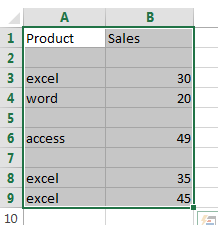#2 go to DATA tab, click Filter command under Sort & Filter group.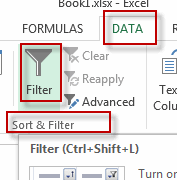#3 the drop-down button should appear in the first cell of your range. Click on this drop down button. de-select the (Blanks) option and then click on the OK button.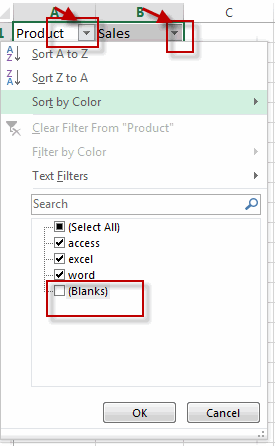#4 you will see that only non-blank cells are displayed in the selected range.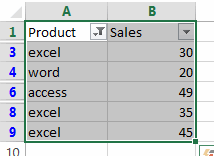#5 then you can select all non-blank cells in the current range and press Ctrl + C shortcuts in your keyboard, and then press Ctrl + V keys to paste the selected cells into a destination cell.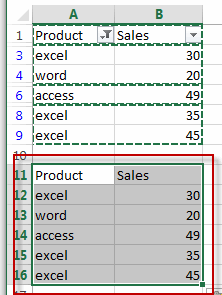## Method2: Copy non-blank Cells with Go To Special Feature

You can also use the Go To Special feature to select all non-blank cells in the selected range of cells. Do the following steps:

#1 select the range of cells that you want to filter all non-blank cells.#2 go to HOME tab, click Find & Select command under Editing group. And select Go To Special from the popup menu list. The Go To Special window will open.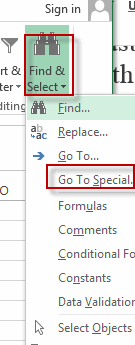#3 select Constants radio button in the Select section under Go To Special dialog. Then click OK button.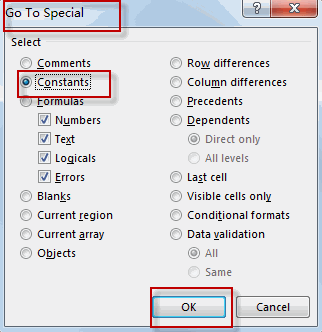#4 all non-blank cells in the selected range are highlighted.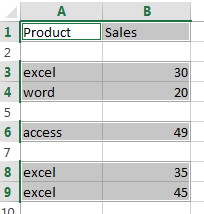#5 you can press Ctrl + C shortcuts to copy cells have been highlighted, and then press Ctrl + V keys to paste the selected cells into a destination cell.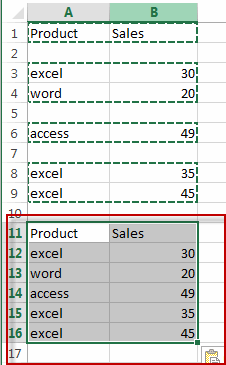## Method3: Copy non-blank Cells with VBA Macro

You can also use an Excel array formula based on the LOOKUP function, the CHOOSE function, the INDEX function, the SMALL function, the IF function, the ROW function and the ROWS function to extract all non-blank cells from a cell. Like this:

`=LOOKUP("xxxxx",CHOOSE({1,2},"",INDEX(A:A,SMALL(IF(\$A\$1:\$A\$9<>"",ROW(\$A\$1:\$A\$9)),ROWS(\$B\$1:B1)))))`

Type this formula into cell B1, and then press Ctrl +Shift +Enter shortcuts to change this formula as array formula. And then drag the AutoFill Handle from Cell B1 to B9.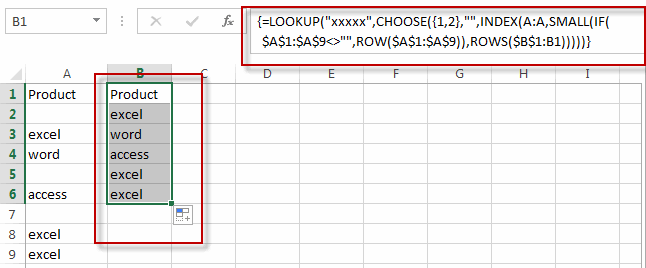All non-blank cells from the range A1:A9 are extracted in the range B1:B9.

## Method4: Copy non-blank Cells with Formula

You can also write an Excel VBA macro to copy and paste all non-blank cells, just do the following steps:

#1 select the range of cells that you want to filter all non-blank cells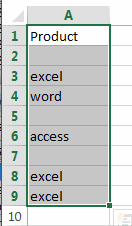#2 open your excel workbook and then click on “Visual Basic” command under DEVELOPER Tab, or just press “ALT+F11” shortcut.#3 then the “Visual Basic Editor” window will appear.

#4 click “Insert” ->”Module” to create a new module.#5 paste the below VBA code into the code window. Then clicking “Save” button.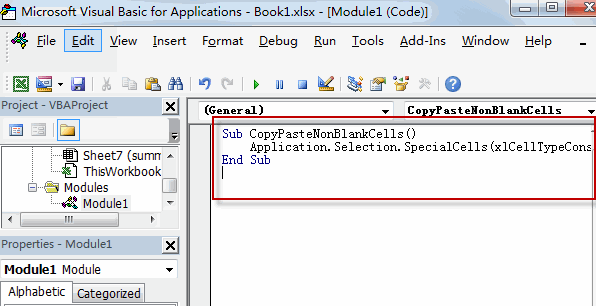```Sub CopyPasteNonBlankCells()
Application.Selection.SpecialCells(xlCellTypeConstants).Copy Destination:=Range("B1")
End Sub```

#6 back to the current worksheet, then run the above excel macro. Click Run button.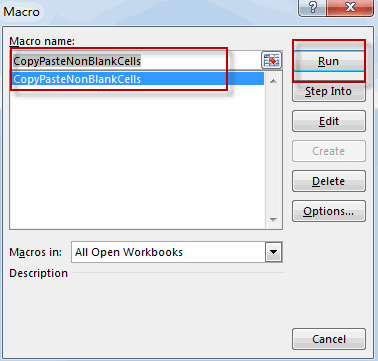#7 let’s see the result.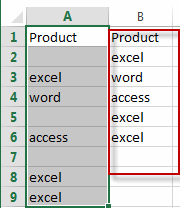### Related Functions

• Excel LOOKUP function
The Excel LOOKUP function will search a value in a vector or array.The LOOKUP function is a build-in function in Microsoft Excel and it is categorized as a Lookup and Reference Function.The syntax of the LOOKUP function is as below:= LOOKUP (lookup_value, lookup_vector, [result_vector])…
• Excel Choose Function
The Excel CHOOSE function returns a value from a list of values. The CHOOSE function is a build-in function in Microsoft Excel and it is categorized as a Lookup and Reference Function.The syntax of the CHOOSE function is as below:=CHOOSE (index_num, value1,[value2],…)…
• Excel INDEX function
The Excel INDEX function returns a value from a table based on the index (row number and column number)The INDEX function is a build-in function in Microsoft Excel and it is categorized as a Lookup and Reference Function.The syntax of the INDEX function is as below:= INDEX (array, row_num,[column_num])…
• Excel ROWS function
The Excel ROWS function returns the number of rows in a cell reference.The syntax of the ROWS function is as below:= ROWS(array)…
• Excel SMALL function
The Excel SMALL function returns the smallest numeric value from the numbers that you provided. Or returns the smallest value in the array.The syntax of the SMALL function is as below:=SMALL(array,nth) …
• Excel ROW function
The Excel ROW function returns the row number of a cell reference.The ROW function is a build-in function in Microsoft Excel and it is categorized as a Lookup and Reference Function.The syntax of the ROW function is as below:= ROW ([reference])….
• Excel IF function
The Excel IF function perform a logical test to return one value if the condition is TRUE and return another value if the condition is FALSE. The IF function is a build-in function in Microsoft Excel and it is categorized as a Logical Function.The syntax of the IF function is as below:= IF (condition, [true_value], [false_value])….

## List all Worksheet Names

This post will guide you how to get a list of all worksheet names in an excel workbook. How do I List the Sheet names with Formula in Excel. How to generate a list of all sheet tab names using Excel VBA Code.

Assuming that you have a workbook that has hundreds of worksheets and you want to get a list of all the worksheet names in the current workbook. And the below will introduce 3 methods with you.

## Get All Worksheet Names Manually

If there are only few worksheets in your workbook, and you can get a list of all worksheet tab names by manually. Let’s see the below steps:

#2 double click on the sheet’s name in the sheet tab. Press Ctrl + C shortcuts in your keyboard to copy the selected sheet.

#3 create a notepad file, and then press Ctrl +V to paste the sheet name.

#4 follow the above steps 2-3 to copy&paste all worksheet names into notepad file.

## Get All Worksheet Names with Formula

You can also use a formula to get a list of all worksheet names with a formula. You can create a formula based on the LOOKUP function, the CHOOSE function, the INDEX function, the MID function, the FIND function and the ROWS function. Just do the following steps:

#1 go to FORMULAS tab, click Name Manager command under Defined Names group. The Name Manager dialog will open.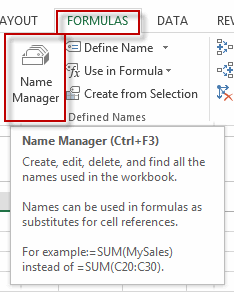#2 click New… button to create a define name, type Sheets in the Name text box, and type the formula into the Refers to text box.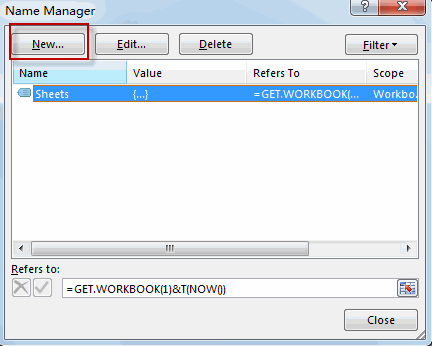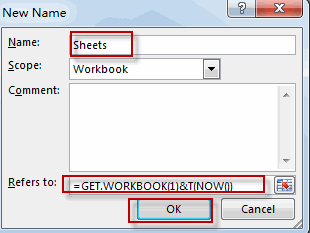`=GET.WORKBOOK(1)&T(NOW())`

#3 Type the following formula into a blank cell and press Enter key in your keyboard, and then drag the autofill handle over others cells to get the rest sheet names.

`=LOOKUP("xxxxx",CHOOSE({1,2},"",INDEX(MID(Sheets,FIND("]",Sheets)+1,255),ROWS(A\$1:A1))))`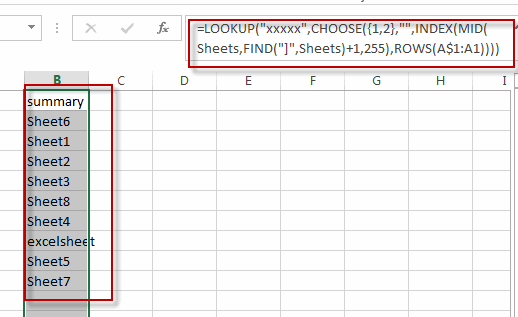You will see that all sheet names have been listed in the cells.

## Get All Sheet Names with Excel VBA Macro

You can also use an Excel VBA Macro to quickly get a list of all worksheet tab names in your workbook. Just do the following steps:

#1 open your excel workbook and then click on “Visual Basic” command under DEVELOPER Tab, or just press “ALT+F11” shortcut.#2 then the “Visual Basic Editor” window will appear.

#3 click “Insert” ->”Module” to create a new module#4 paste the below VBA code into the code window. Then clicking “Save” button.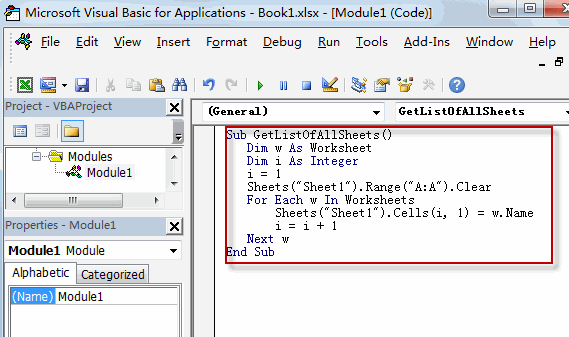```Sub GetListOfAllSheets()
Dim w As Worksheet
Dim i As Integer
i = 1
Sheets("Sheet1").Range("A:A").Clear
For Each w In Worksheets
Sheets("Sheet1").Cells(i, 1) = w.Name
i = i + 1
Next w
End Sub```

#5 back to the current worksheet, then run the above excel macro. Click Run button.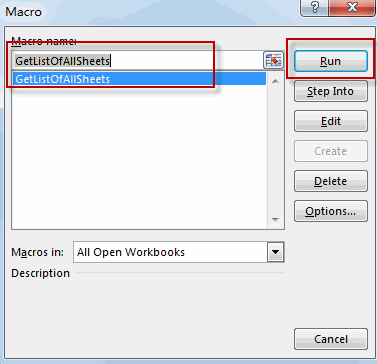#6 Let’s see the result.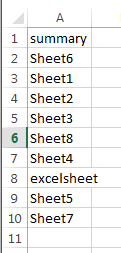### Related Functions

• Excel MID function
The Excel MID function returns a substring from a text string at the position that you specify.The syntax of the MID function is as below:= MID (text, start_num, num_chars)…
• Excel LOOKUP function
The Excel LOOKUP function will search a value in a vector or array.The LOOKUP function is a build-in function in Microsoft Excel and it is categorized as a Lookup and Reference Function.The syntax of the LOOKUP function is as below:= LOOKUP (lookup_value, lookup_vector, [result_vector])…
• Excel Choose Function
The Excel CHOOSE function returns a value from a list of values. The CHOOSE function is a build-in function in Microsoft Excel and it is categorized as a Lookup and Reference Function.The syntax of the CHOOSE function is as below:=CHOOSE (index_num, value1,[value2],…)…
• Excel INDEX function
The Excel INDEX function returns a value from a table based on the index (row number and column number)The INDEX function is a build-in function in Microsoft Excel and it is categorized as a Lookup and Reference Function.The syntax of the INDEX function is as below:= INDEX (array, row_num,[column_num])…
• Excel ROWS function
The Excel ROWS function returns the number of rows in a cell reference.The syntax of the ROWS function is as below:= ROWS(array)…
• Excel FIND function
The Excel FIND function returns the position of the first text string (sub string) within another text string.The syntax of the FIND function is as below:= FIND(find_text, within_text,[start_num])

## Replacing Cell References in Multiple Cells (Formulas)

This post will guide you how to replace a cell reference in multiple cells in excel. How do I replace Cell or Sheet reference in copied formulas in your worksheet in excel. How to quickly find and replace a cell reference in excel formula. How to replace references in formula with another Cell reference or value in excel. And this post will explain that how to find and replace part of formula in all cells in excel.

## Replacing Cell References

Assuming that you have applied the following formula in cell C1, and then copied this formula over other cells.

` =DATE(\$B\$1,1,1)+CHOOSE(WEEKDAY(DATE(B1,1,1),2),0,6,5,4,3,2,1)`

If you want to change part of formula for all copied formula, for example, changing one cell reference \$B\$1 to \$A\$1, you can use the Find and Replace feature to achieve it. Just do the following steps:

#1 select the range of cells that you have applied this formula.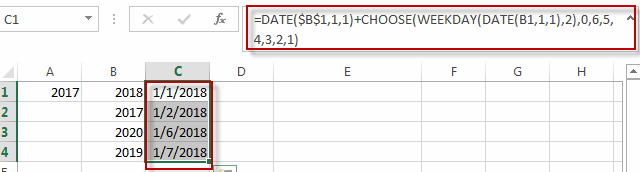#2 go to HOME tab, click Find & Select command under Editing group, then select Replace… from the drop-down menu list, the Find and Replace dialog will appear.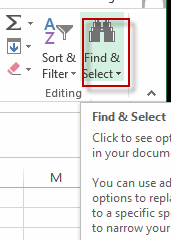#3 type \$B\$1 into the text box of Find what, and type \$A\$1 into the text box of Replace with box. Then click Options<< button.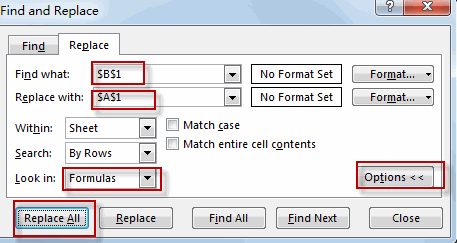#4 select Formulas from the Look in list box, then click Replace All button.

#5 click OK button.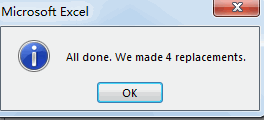#6 let’s see the result.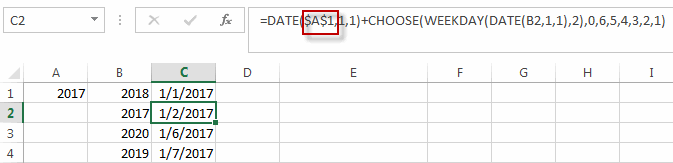You will see all cell references have been replaced with another cell reference in all copied formulas.

### Related Functions

• Excel DATE function
The Excel DATE function returns the serial number for a date.The syntax of the DATE function is as below:= DATE (year, month, day)…
• Excel Choose Function
The Excel CHOOSE function returns a value from a list of values. The CHOOSE function is a build-in function in Microsoft Excel and it is categorized as a Lookup and Reference Function.The syntax of the CHOOSE function is as below:=CHOOSE (index_num, value1,[value2],…)…

## Get the First Monday of a Given Year

This post will guide you how to get the first Monday of a given year or date in excel. How do I calculate the first Monday of a specific year in excel. How to determine the first Monday in a given year date with a formula in excel. How to find and get the date of the first Monday of a given year date in excel.

## Get the First Monday of a Given Year

To calculate the first Monday of the year or given any date, you can create a new complex formula based on the DATE function, the YEAR function and the WEEKDAY function.

Assuming that you have a date list in the range of cells B1:B4 in your current worksheet, and if you want to get the first Monday for the year date in each cell, and you can write down one formula as below:

`=DATE(YEAR(B1),1,8)-WEEKDAY(DATE(YEAR(B1),1,6))`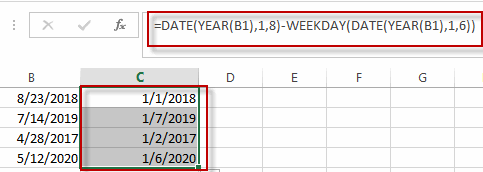Type this formula into the formula box of a blank cell such as: C1, then press Enter key in your keyboard, and drag the AutoFill Handler over other cells to apply this formula.

You can also use another formula based on the DATE function, the CHOOSE function and the WEEKDAY function to create the same result. Like this:

`=DATE(B1,1,1)+CHOOSE(WEEKDAY(DATE(B1,1,1),2),0,6,5,4,3,2,1)`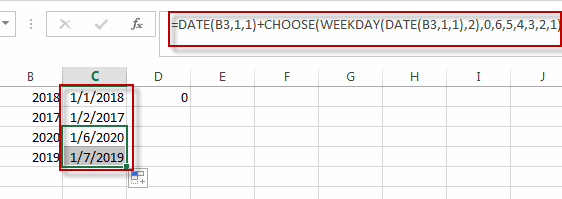### Related Functions

• Excel WEEKDAY function
The Excel WEEKDAY function returns a integer value representing the day fo the week for a given Excel date and the value is range from 1 to 7.The syntax of the WEEKDAY function is as below:=WEEKDAY (serial_number,[return_type])…
• Excel DATE function
The Excel DATE function returns the serial number for a date.The syntax of the DATE function is as below:= DATE (year, month, day)…
• Excel YEAR function
The Excel YEAR function returns a four-digit year from a given date value, the year is returned as an integer ranging from 1900 to 9999. The syntax of the YEAR function is as below:=YEAR (serial_number)…
• Excel Choose Function
The Excel CHOOSE function returns a value from a list of values. The CHOOSE function is a build-in function in Microsoft Excel and it is categorized as a Lookup and Reference Function.The syntax of the CHOOSE function is as below:=CHOOSE (index_num, value1,[value2],…)…

## Excel Nexted IF Functions (Statements) Tutorial (15 IF Formulas examples)

This tutorial will guide you how to use nested Excel IF function  (include multiple If statements in excel formula) with syntax and provide about 15 nested IF formula examples with the detailed explanation in Microsoft excel.

## Description

The Excel IF function perform a logical test to return one value if the condition statement is TRUE and return another value if the condition statement is FALSE. The IF function is a build-in function in Microsoft Excel and it is categorized as a Logical Function.

The excel if function just only test one condition and if you want to deal with more than one condition and return different actions depending on the result of the tests,  then you need to include several IF statements (functions) in one excel IF formula, these multiple IF statements are also called Excel Nested IF formula(Nested IFs). It’s also similar with IF-THEN-ELSE statement.

The nested IF function is formed by multiple if statements within one Excel if function. This excel nested if statement makes it possible for a single formula to take multiple actions.

The most recent versions of Excel (Excel 2016, Excel 2013, Excel 2010 and Excel 2007) allow 64 IF functions (statements) in one formula, and it was only possible up to 7 nested IF functions (statements) in Excel 2003 and lower version.

## Syntax

The syntax of Nested IF function is as below:

`=IF(Condition_1,Value_if_True_1,IF(Condition_2,Value_if_True_2,Value_if_False_2))`

Where the Nested IF function argument is:

Condition_1 –  The condition that you want to test  in the first IF statement.

Value_if_True_1 – The value that is returned if first IF statement is True. If the condition_1 return False, then move into the next IF function.

Condition_2 – The condition that you want to test in the second IF statement.

Value_if_True_2 – The value that is returned if second IF statement is True.

Value_if_False_2 – The value is returned if second IF statement is False.

This is equivalent to the following IF THEN ELSE statement:

```IF Condition_1 THEN
Value_if_True_1
ELSEIF Condition_2
Value_if_True_2
ELSE
Value_if_False_2
END IF```

## Examples of Nested IF function (Statement) in Excel

The below examples will show you how to use Excel Nested IF function with the detailed explanation of their syntax and logic.

Example 1#  The most basic Nested IF function with one level of nesting

If you want to write a nested if function to test the following calculation logic for assigning value in the cell A1.

```IF A1 =="excelhow" THEN
return "excel"
ELSEIF A1 == "excelhow.net" THEN
return "MS excel"
ELSE
return "MS"
END IF```

we can write a nested IF function based on the above logic as follows:

`=IF(A1="excelhow", "excel", IF(A1="excelhow.net","MS excel","MS"))`

In above Nested IF formula, the nested if function is shown with bold style and it is inside the outer IF function. we can see that if A1 is not equal to the “excelhow“, then the second nested  IF function will be test. and if second IF condition statement return FALSE, then the entire IF function will return “MS” value.

Example 2#  The Nested IF function with two levels of nesting

Assuming that you want to test more than one condition statement in the above nested if function, add one condition to test if the value of the cell A1 reference is equal to “www.excelhow.net” , If TRUE, then return “Microsot excel“.

The calculation Logic is as below:

```IF A1 =="excelhow" THEN
return "excel"
ELSEIF A1 == "excelhow.com" THEN
return "MS excel"
ELSEIF A1 == "www.excelhow.net" THEN
return "Microsoft excel"
ELSE
return "MS"
END IF```

we can add one more IF statement inside the second IF function in the above excel nested if formula in example1. let’s see the below nested if function with tow level nesting:

`=IF(A1="excelhow", "excel", IF(A1="excelhow.com","MS excel",IF(A1="www.excelhow.net","Microsoft excel","MS")))`

In the above nested excel IF formula, the first nested if function is marked with red color, and the second nested excel if function is marked with blue color.

If the both first and second conditions are False and the third IF condition will be check, IF A1 is equal to “www.excelhow.net” , then return “Microsoft excel“, or the entire nested IF formula will return “MS“.

Example 3#  Describes the each IF function contained in the nested IF function

​We will use one typical example of excel nested if function to describe each IF function included in the nested if function.

Assuming that you need to assign a grade based on a score with the following test conditions:

Let’s write a nested if function based on the above logic as follows:

`=IF(A1>=80, "excellent", IF(A1>=60, "good", IF(A1>0, "bad", "no valid score")))`

For the above excel if formula, lets describe it for each IF function statement.

1# IF Cell A1 is greater than or equal to 80, then the formula will return “excellent” or move to the second If function.

2# If Cell A1 is greater than or equal to 60, then the formula will return  “good” or move to the third IF function

3# IF Cell A1 is greater than 0, then the formula will return “bad”, or the IF function will return “no valid score”.

Example 4#  Describes each If function in the excel Nested IF Statement (another simple example of if function)

Let’s describe the below Nested IF Function example:

`=IF(A1<=6,60, IF(A1<=8,80,IF(A1<=10,100,200)))`

a) If cell A1 is equal to 6 or less than 6, then return value 60 in cell C1. Let’s see below screenshot.

b) If Cell A1 is greater than 6 and less or equal to 8, then retrun value 80 in Cell C1.

c) If cell A1 is greater than 8 and less than or equal to 10, then return value 100 in cell C1.

d) If cell A1 is greater than 10 , then the Nested if function will return the last value “200”in cell C1.

Example 5#  Excel Nested IF function with arithmetic operator (+, -, * , /)

Assuming that you want to write a Nested If function to reflect the following logic tasks:

a) IF Cell A1 is less than 10, then multiply by 10.

b) IF Cell A1 is greater than or equal to 10 but less than 20, then add 20

c) IF Cell A1 is greater than or equal to 20 but less than 30, then minus 20

d) IF Cell A1 is greater than or equal to 30 but less than 50, then divided by 20

The nested IF formula is as follows:

`=IF(A1<10,A1*10,IF(A1<20,A1+20,IF(A1<30,A1-20,IF(A1<50,A1/20))))`

a1) if Cell A1 is less 10 (A1=5), then the first If condition matched and will take multiply action, A1 * 10=5*10=50, so it will return 50 in the cell C1

b1) if Cell 10<=A1<20 (A1=15), then the second if condition matched and will take add action, A1+20=15+20=35, so it will return 35 in the cell C1.

c1) if Cell 20<=A1<30(A1=25), then the third if condition matched and will take minus action, A1-20=25-20=5, so it will return 5 in the cell C1.

d1) if Cell 30<=A1<50 (A1=35), then the forth if condition matched and will take divide action, A1/20=35/20=1.75, so it will return 1.75 in the cell C1.

Example 6#  Excel Nested IF function with logical function –AND

Assuming that you need a nested if function to reflect the following logic:

a) IF A1+B1 is less than 10, then return 10

b) IF A1+B1 is greater than 10 but less than or equal to 20, then return 20

c) IF A1+B1 is greater than 20 but less than or equal to 30, then return 30.

d) IF A1+B1 is greater than 30, then return 200.

Let’s write the following nested IF formula in the cell C1:

`=IF(A1+B1<10,10, IF(A1+B1<=20,20, IF(A1+B1<=30,30,200)))`

The above formula just use basic nested IF function syntax, we also can use logic function to re-write it, the nested if formula with AND function is as follows:

`=IF((A1+B1)<10,10,IF(AND((A1+B1)>10,(A1+B1)<=20),20, IF(AND((A1+B1)>20,(A1+B1)<=30),30,200)))`

The above nested IF formula combined with two AND function.

In the second IF Statement, AND((A1+B1)>10,(A1+B1)<=20) will check if 10<A1+B1<=20, If TRUE, then the formula will return 20.

In the third IF Statement, AND((A1+B1)>20,(A1+B1)<=30) will check if 20<A1+B1<=30, If TRUE, then the formula will return 30.

Example 7# Excel Nested IF function with logical function –OR

Assuming that you need a nested if function to reflect the following logic:

a) IF Cell A1=5, return A1/B1

b) IF Cell A1=10, return A2/B2

c) IF Cell A1=15 or A1=20, return A3/B3

In Cell C1, we can write the below nested if formula based on the above conditions.

`=IF(A1=5,A1/B1,IF(A1=10,A2/B2,IF(OR(A1=15,A1=20),A3/B3)))`

One OR function be used in the above excel nested if function, it will check if A1=15 or A1=20, if TRUE, then return A3/B3.

Example 8#  Excel nested if function with text and logical function AND

Wrote a nested if function with text to reflect the following logic:

a) If Cell A1=”E” and Cell B1=5, then return “Excel”

b) If Cell A1=”P” and Cell B1=3, then return “PPT”

c) If Cell A1=”W” and Cell B1=4, then return “Word”

d) Else return “Access”

In Cell C1, try to enter into the following excel nested If formula with AND function:

`=IF(AND(A1="E",B1=5),"Excel", IF(AND(A1="P",B1=3),"PPT", IF(AND(A1="W",B1=4),"Word","Access")))`

Example 9#  Excel nested if function with ISBLANK function and logical function AND

a) If you want to wrote a nested if function with ISBLANK function and logical function AND to reflect the following logic:

b) If both Cell A1 and Cell B1 are empty, then return “”

c) If only Cell A1 is empty, then return B1-today()

d) If both two cells A1 and B1 are not empty, then return “excel” string.

In Cell C1, use the following excel nested If formula with ISBLANK and AND function:

`=IF(AND(ISBLANK(A1),ISBLANK(B1)),"",IF(ISBLANK(A1),B1-TODAY(),"excel"))`

Example 10#  Using nested IF functions to check grade level based on student’s score(multiple IF statements)

The logic is as below:

We will write a nested If function that reflect the above logic, and will check if the score is below 50, If TRUE, it is considered as “Fail”. If FALSE, move into the next IF statement to test if the score is between 51 and 60 and it is considered as “Grade C”. If False, we will move into another IF statement to check if the score is between 61 and 80, IF True and it is considered as “Grade B”. If FASLSE, just check the rest conditions.

We can use a nested if formula as follows:

`=IF(B2<=50,"Fail",IF(AND(B2>=51, B2<=60), "Grade C", IF(AND(B2>=61, B2<=80),"Grade B",IF(AND(B2>=81,B2<=95),"Grade A", "Grade A+"))))`

Example 11#  Nested IF function for checking two Empty Cells

Let’s see the below image a product table of a company (need to create an excel table firstly):

a) If we need to check both “Price” cell and “Quantity” cell are empty, If True, then return empty. If the only “price” cell is empty only, IF True, return empty.

b) If the only “Quantity” cell is empty, IF True, return empty.

c) If both “price” and “Quantity” are not empty, then return multiply Price * Quantity as subtotal value.

So To check both “Price” and Quantity cells, we can use table header name as condition variable to test each Price cells or Quantity cells, so we can write the nested if formula as follows:

`=IF([Price]="","",IF([Quantity]="","",[Price]*[Quantity]))`

Just using the above excel if formula in the subtotal cells, the formula will check the first IF statement if Price Cell is empty, IF TRUE, then will return empty (“”) in the subtotal cell. IF FALSE, then move to the next IF statement and so on. Last, IF neither cell is empty, then will return the value of multiply [Price]*[Quantity] in subtotal cell.

Of course, we can use another nested if function to achieve the above calculation logic (easy to understand).

`=IF(ISBLANK(B2),"",IF(ISBLANK(C2),"", B2*C2))`

OR

`=IF(B2="","",IF(C2="","", B2*C2))`

## Nested IF Functions Order

There is one important thing that need us keep in mind when write Excel Nested IF Function, it is the order of nested IF function. It can nested up to 64 If statements, and how to order multiple IF condition statements, it is key point. Or the wrong result will be returned. The point is that excel nested if function will test the first if condition in the order, once any condition is met, and the subsequent if conditions will not be checked.

So let’s remember the below rules while writing excel nested if function:

• The most important condition First or Harder Test First

Let’s see the below example what it means:

Example 12#

There are two test conditions in the following excel nested if function:

`=IF(B1>=90,"excellent",IF(B1>=60,"good","bad"))`

When using this formula in the cell B3, If the amount in cell B1 is 95, then “excellent” would be returned. because it is greater than 90. And the second IF condition will not be evaluated.

However, if the order of nested if statment are reversed as follows:

`=IF(B1>=60,"good",IF(B1>=90,"excellent","bad"))`

The above formula would check for the condition B1>=60 first, if the amount in cell B1 is 95,  then the value “good” would be returned in cell B3. Because the Cell B1 match the first test condition, and it will not check the second if condition and will return the incorrect result.

## Nested IF Function Alternatives

To make your excel formulas more efficiency and fast, you can try to use the following alternatives to excel nested if function.

1)
Excel nested if function can be easily replaced with the VLOOUP, Lookup, INDEX/MATCH or CHOOSE functions.

Example 13#  Use VLookup function instead of nested IF function

Nested IF function:

`=IF(D1=39,"excelhow.net", IF(D1=45,"two",IF(D1=34,"google.com","")))`

Vlookup function:

`=VLOOKUP(39,A1:B3,2,FALSE)`

Example 14#  Use CHOOSE function instead of nested if function

Nested If unction:

`=IF(B1>=60,"good","bad")`

Using CHOOSE function as follows:

`=CHOOSE(IF(B1<60,1,2),"bad","good")`

2) Use IFS instead of nested if function

3) Use the CONCATENATE function or the concatenate operator (&).

Example 15#

Nested IF function:

`=IF(B1="A","excellent",IF(B1="B","good","bad"))`

Using CONCATENATE function as follows:

`=CONCATENATE(IF(B1=”A”,”excellent”,””),IF(B1=”B”,”good”,””),IF(B1=”C”,”bad”))`

Question1: Is there any tool to help write Excel formulas and nested Ifs?

This is an Excel formula with nested IF statements:

`=IF((B2="East"),4,IF((B2="West"),3,IF((B2="North"),2,IF((B2="South"),1,""))))`

To essentially accomplish this:

```If cell B2 = "East"
return "4"
ElseIf cell B2 = "West"
return "3"
ElseIf cell B2 = "North"
return "2"
ElseIf cell B2 = "South"
return "1"
Else
return ""```

Can Excel formulas be written in such a “more readable” manner and converted to the official syntax? Is there any tool to help write Excel formulas?

Answer: Excel Formula Formatter add-in by Rob van Gelder, mentioned at Daily Dose of Excel.

Excel’s formula bar ignores line feeds and white space, so you can Alt+Enter and spacebar to format the formulas however you like. I’ve tried it and I quickly stopped doing it. Too much spacebar-ing, especially if you need to edit.

Question2: I am working on an excel file, and i am trying to use a nested if formula to achieve what i would like.

i have two columns:A B.  And the condition is this: if the value in a2=a3, then check if the minus of b2 and b3 is certain value, and if it is, put a yes, else put a no. this will iterate till the end of the excel file.

so far here is what i have. not sure how to use the excel formulas. any help is much appreciated.

`if(a2=a3,b2-b3=5 or b2-b3=-5 or b2-b3=20 or b2-b3=-20, "yes", "no")`

Answer: you should be able to use the OR function within your nested if formula to test for “B2-B3=5,B2-B3=-5,B2-B3=20,B2-B3=-20” as follows:

`=IF(A2=A3,IF(OR(B2-B3=5,B2-B3=-5,B2-B3=20,B2-B3=-20),"yes","no"),"no")`

### Related Functions

• Excel IF function
The Excel IF function perform a logical test to return one value if the condition is TRUE and return another value if the condition is FALSE….
• Excel And function
The Excel AND function returns TRUE if all of arguments are TRUE, and it returns FALSE if any of arguments are FALSE…
• Excel Concat Function
The excel CONCAT function combines 2 or more strings or ranges together. The syntax of the CONCAT function is as below: =CONCAT (text1,[text2],…) …
• Excel Vlookup Function
The Excel VLOOKUP function lookup a value in the first column of the table and return the value in the same row based on index_num position.The syntax of the VLOOKUP function is as below:= VLOOKUP (lookup_value, table_array, column_index_num,[range_lookup])…
• Excel Choose Function
The Excel CHOOSE function returns a value from a list of values. The CHOOSE function is a build-in function in Microsoft Excel and it is categorized as a Lookup and Reference Function.The syntax of the CHOOSE function is as below:=CHOOSE (index_num, value1,[value2],…)…
• Excel ISBLANK function
The Excel ISBLANK function returns TRUE if the value is blank or null.The syntax of the ISBLANK function is as below:= ISBLANK (value)…

## Excel Choose Function

This post will guide you how to use Excel CHOOSE function with syntax and examples in Microsoft excel.

### Description

The Excel CHOOSE function returns a value from a list of values.

The CHOOSE function is a build-in function in Microsoft Excel and it is categorized as a Lookup and Reference Function.

The CHOOSE function is available in Excel 2016, Excel 2013, Excel 2010, Excel 2007, Excel 2003, Excel XP, Excel 2000, Excel 2011 for Mac.

### Syntax

The syntax of the CHOOSE function is as below:

=CHOOSE (index_num, value1,[value2],…)

Where the CHOOSE function arguments are:
index_num -This is a required argument. Specify the position number in the list of values.
Value1,[value2] – This is a required argument.  A list of one or more values that you want to return
Note: The index_num value must be a number between 1 and 29.
If index_num value is less than 1 or greater than the length of the value list, the CHOOSE function will return #VALUE! Error value.

### Example

The below examples will show you how to use Excel CHOOSE Lookup and Reference Function to return a value from a value list based on a position value.

#1 To get the second value in the value list in B1 Cell, just using the following excel formula: =CHOOSE(2,A1,A2,A3)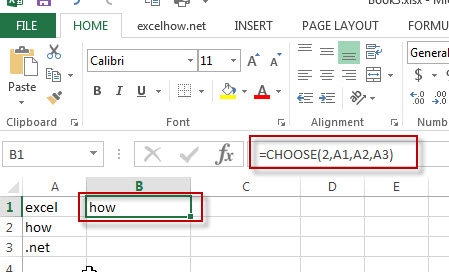### More Excel Choose Function Examples

• Get the First Monday of a Given Year
To calculate the first Monday of the year or given any date, you can create a new complex formula based on the DATE function, the YEAR function and the WEEKDAY function……
• List all Worksheet Names
Assuming that you have a workbook that has hundreds of worksheets and you want to get a list of all the worksheet names in the current workbook. And the below will introduce 3 methods with you..…
• Copy and Paste Only Non-blank Cells
If you want only copy non-blank cells in a range in Excel, you need to select the non-blank cells firstly, then press Ctrl +C keys to copy the selected cells. So how to only select all non-blank cells in the selected range in your worksheet..…